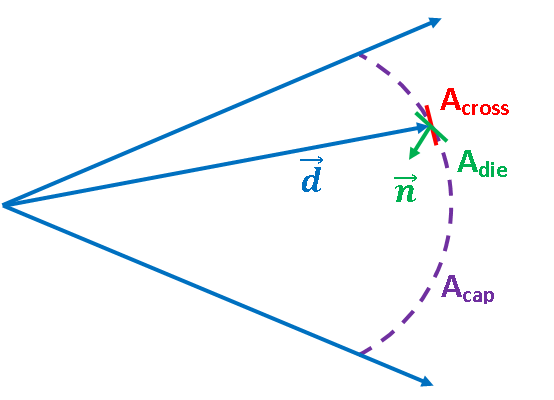Spray cooling is a complicated process with multiple factors at play. One such factor is the Heat Transfer Coefficient (HTC), which itself depends on many variables such as spray shape, coolant flow rate, spray pressure, mold temperature, spray angles, and spray distance. In FLOW-3D CAST, a die spray model was developed in collaboration with Audi, where the HTC was computed by a series of spatial dependence factor functions in order to capture the effects of all these variables. However, it is often the case that the user doesn’t know the value of these dependence factor functions. A new, simpler way to calculate the HTC has been developed in FLOW-3D CAST v5.0, which is based on known or easy-to-obtain spray medium properties. In this blog, I will talk about the new HTC calculation method followed by an example and a validation.

## Basing Heat Transfer Coefficients on Spray Medium Properties

Practically, the spray cooling HTC can be computed from spray medium properties based on energy exchange. Assuming the nozzle produces a uniform and conical spray, as illustrated in the simplified 2D version below, the HTC value can be expressed as$latex HTC=~\alpha \cdot \rho \cdot \frac{{\dot{V}}}{{{{A}_{{cap}}}}}\cdot \frac{{\vec{n}\cdot \vec{d}}}{{\left| {\vec{d}} \right|}}\cdot \frac{{\left[ {\frac{{{{T}_{{die}}}-{{T}_{{spray}}}}}{{{{\tau }_{T}}}}\cdot L+{{C}_{p}}\cdot \left( {{{T}_{{evapo}}}-{{T}_{{spray}}}} \right)} \right]}}{{{{T}_{{die}}}-{{T}_{{spray}}}}}$

where

•  α is the efficiency coefficient
•  ρ is the density of the spray medium
• $latex \dot{V}$ is the nozzle volume flow rate
•  Acap is the spray cone cap area at spray distance d
•  Adie is the die surface element area
•  Across is the surface element cross area
•  τT is the latent heat release temperature range
•  L is the evaporation latent heat
•  Cp is the specific heat
•  Tdie is the die surface temperature
•  Tevapo is the spray medium evaporation temperature
•  Tspray is the spray medium temperature

This equation shows us that the dependencies on the die temperature, the spray distance and the spray angles are included in the calculations, making the calculations more realistic and accurate.

Note that the equation is valid when the die temperature is within [TevapoTevapo +τT]. When the die temperature is below Tevapo, the equation is reduced to:

$latex HTC=~\alpha \cdot \rho \cdot \frac{{\dot{V}}}{{{{A}_{{cap}}}}}\cdot \frac{{\vec{n}\cdot \vec{d}}}{{\left| {\vec{d}} \right|}}\cdot {{C}_{p}}$

When the die temperature is above Tevapo +τT, due to the advection of the air and spray medium moisture, the HTC is kept as a constant value.

## Sample Simulation

This thermal die cycling simulation demonstrates the capability of the spray cooling model with the new HTC calculator, and the importance of accurately simulating the die spraying process. The animation below shows the simulation results of the production of a vehicle structural part with large dimension and thin wall thickness. In this example, the simulation is limited to the ejector part of the die.

## Validation

A comparison between the measured data (courtesy of Audi AG) and the computed value at the center of a spray cone as a function of temperature shows that the HTCs computed match well with the measurements.HTCs based on spray medium properties provide an easy and convenient way for defining accurate HTCs for the spray cooling process and allows our users to avoid difficult and expensive measurements.Subscribe to the Blog
Privacy *